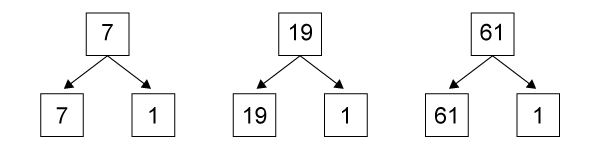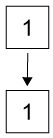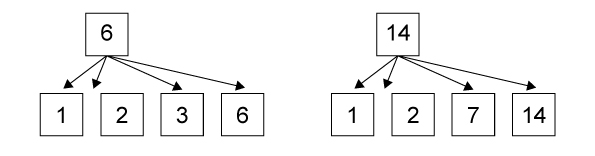# Types of Numbers in Maths With Examples

Numbers play a very important part in our lives. We use numbers to tell the time, for the sake of measurement, to weigh objects, and even to count money. We introduce number digits to kids gradually, firstly single digits, and then tens, hundreds, thousands, and so on.

Numbers are identified in different types such as decimal numbers, fractions, and other such categories, for the sake of simplifying the concept and offering clarity to kids. Through this article, let us elaborate on the different kinds of numbers and what each of them signifies.### Natural Numbers

As the word suggests, natural numbers are any counting number that consists of all positive numbers from 1 to infinity. This set does not include zero or negative numbers such as (Example: -1, -19, -13).  Another condition that it abides by, is that the numbers have to be integers, and not fractions or decimal numbers. Basically, an integer is defined as a whole number (Example: 12, 99, 1000) are all integers, but (Example: ½, ¾, ¼) are not considered integers, because they are fractions, hence not a natural number.

Struggling to teach your little one natural numbers? Try out the Mathseeds e-learning platform, where children get to interact with their favorite mascot ‘Eggy’, and learn Eggy Numbers from the comfort of their homes. Mathseeds is available for subscription in the UAE, including the GCC counterparts.

### Whole Numbers

Whole numbers are a set of natural numbers including zero. They exclude all fractions, decimals, or negative numbers. The only differentiating factor between natural numbers and whole numbers is that whole numbers include zero ‘0’, while natural numbers don’t.

### Integers

An integer is basically any number that is not a fraction, a decimal, or a percentage. Other than these three exceptions, all numbers are integers. All positive numbers are integers (Example: 10, 74, 1001, 678), all negative numbers are also integers (-133, -792, -2), and zero (0) is also an integer. So, zero to infinity and all negative numbers are integers.

### Rational Numbers

The word rational was borrowed from the term ‘ratio’. Mainly rational numbers are in the form of p/q, which means p is the integer and q ≠ 0. These numbers are in the form of a fraction to be included in the rational number category.

Let us see some examples of rational numbers;

• 30/1 (here both numbers are integers, hence it is a rational number).
• 0/1 (here where p is 0 and q is 1, so the fraction can be considered as a rational number).
• -3/-6 (both p and q are negative integers, they are therefore considered rational numbers. This is also called a positive rational number, as the negatives in the numerator and denominator get cancelled out and give a positive answer).
• -12/7 (the numerator is a negative integer and the denominator is a positive integer, but since both qualify as integers, this example is also a rational number. The scenario is called a negative rational number, since the numerator denotes a negative sign, hence the answer will also be negative).

### Even Numbers

A number that can be exactly divided by 2 is called an even number. This is the simplest to remember and children enjoy segregating even numbers as a Math exercise. Some even numbers are; 2, 4, 10, 24, 32, 68, 90, etc. Even 0 qualifies as an even number, because, if zero is divided by any number the answer is zero itself and thus, it is an even number. Let us remember, any number which is divisible by 2 and generates a remainder of 0 is called an even number.

### Odd Numbers

On the contrary, an odd number is any number that is not divisible by 2 wholly, where the remainder comes out as zero. In fact, when you try to divide the odd number by 2, the remainder is always 1. Try it out if you have to! Some examples of odd numbers are; 13, 7, 29, 55, 97, and so on. Children can start learning about odd numbers via gamified experiences on the Mathseeds App. This platform is trusted by over 20 million users and is a favorite amongst Math enthusiasts in the Middle East, including Dubai, and other regions of the world.

### Even Numbers

A number that can be exactly divided by 2 is called an even number. This is the simplest to remember and children enjoy segregating even numbers as a Math exercise. Some even numbers are; 2, 4, 10, 24, 32, 68, 90, etc. Even 0 qualifies as an even number, because, if zero is divided by any number the answer is zero itself and thus, it is an even number. Let us remember, any number which is divisible by 2 and generates a remainder of 0 is called an even number.

### Odd Numbers

On the contrary, an odd number is any number that is not divisible by 2 wholly, where the remainder comes out as zero. In fact, when you try to divide the odd number by 2, the remainder is always 1. Try it out if you have to! Some examples of odd numbers are; 13, 7, 29, 55, 97, and so on. Children can start learning about odd numbers via gamified experiences on the Mathseeds App. This platform is trusted by over 20 million users and is a favorite amongst Math enthusiasts in the Middle East, including Dubai, and other regions of the world.

### Prime Numbers

A prime number is a number that has two factors, out of which, ‘1’ must be a factor and the other is the number itself. Let us see some examples below:Wondering if 1 is a prime number itself?1 is not a prime number, since it has only ‘one’ factor, therefore, not fulfilling the above criteria.

### Composite Numbers

A composite number has more than two factors and is just the opposite of prime numbers. Let us see some examples of composite numbers.As we can we in the above examples, the composite numbers have multiple factors.

Conclusion: Children may find learning all these different classifications of numbers a little overwhelming. However, we suggest you introduce them in stages so that each concept is understood with clarity. Follow the lesson with lots of practice and problem-solving questions, so that there is no chance for confusion.